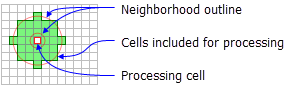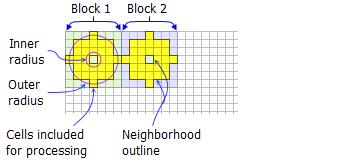# NbrAnnulus

Mit der Spatial Analyst-Lizenz verfügbar.

## Zusammenfassung

Defines an annulus neighborhood which is created by specifying an inner and outer circles' radii in either map units or number of cells.

## AbbildungNbrAnnulus neighborhood example for the FocalStatistics function (inner radius 1 cell, outer radius 3 cells).NbrAnnulus neighborhood example for the BlockStatitistcs function (inner radius = 1 cell, outer radius = 3 cells).

## Auswertung

Tools that use neighborhood annulus object: Block Statistics, Focal Statistics, Point Statistics, and Point Density.

##### Lizenz:

This class is also available if you have an Image Analyst extension license, but only for the Focal Statistics tool.

The annulus shape is comprised of two circles, one inside the other to make a donut shape. Cells with centers that fall outside the radius of the smaller circle but inside the radius of the larger circle will be included in processing the neighborhood; therefore, the area that falls between the two circles constitutes the annulus neighborhood.

When the annulus object is used and the innerRadius or the outerRadius are not specified, then default values are provided.

The innerRadius must be less than or equal to the outerRadius.

The radius is identified in cells or map units, measured perpendicular to the x- or y-axis. When the radii is specified in map units, they are converted to radii in cell units. The resulting radii in cell units produces an area that most closely represents the area calculated by using the original radii in map units. Any cell center encompassed by the annulus will be included in the processing of the neighborhood.

## Syntax

`NbrAnnulus ({innerRadius}, {outerRadius}, {units})`
 Parameter Erklärung Datentyp innerRadius The inner radius of an annulus neighborhood.(Der Standardwert ist 1) Double outerRadius The outer radius of an annulus neighborhood.(Der Standardwert ist 3) Double units Defines the units of the neighborhood.CELL —The unit of measurement is in cells.MAP —The units are in map coordinates.(Der Standardwert ist CELL) String

## Eigenschaften

 Eigenschaft Erklärung Datentyp innerRadius(Lesen und schreiben) The inner radius of the annulus neighborhood. Double outerRadius(Lesen und schreiben) The outer radius of the annulus neighborhood. Double units(Lesen und schreiben) Defines the units of the neighborhood. String

## Codebeispiel

NbrAnnulus example 1 (Python window)

Demonstrates how to create a NbrAnnulus class and use it in the BlockStatistics tool within the Python window.

``````import arcpy
from arcpy import env
from arcpy.sa import *
env.workspace = "C:/sapyexamples/data"
outNbrA = BlockStatistics("block", NbrAnnulus(1, 2, "CELL"))
outNbrA.save("C:/sapyexamples/output/blstatnbra2")``````
NbrAnnulus example 2 (stand-alone script)

Implements the BlockStatistics tool using the NbrAnnulus class.

``````# Name: NbrAnnulus_Ex_02.py
# Description: Uses the NbrAnnulus object to execute BlockStatistics tool.
# Requirements: Spatial Analyst Extension

# Import system modules
import arcpy
from arcpy import env
from arcpy.sa import *

# Set environment settings
env.workspace = "C:/sapyexamples/data"

# Set local variables
inRaster = "block"

# Create the Neighborhood Object